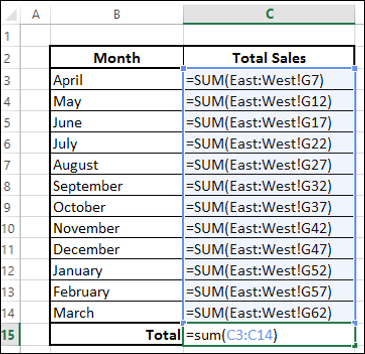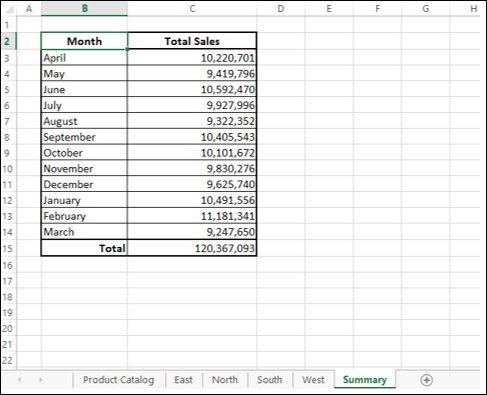# Working with Multiple Sheets

In certain situations, you might have to

• Set up workbooks in which several worksheets have a similar format or structure.
• Get information for these worksheets from another worksheet.
• Summarize the results from these worksheets into a summary worksheet.

For example, you might have to track sales information region wise and month wise in separate worksheets. The price of each product is taken from the product catalog that is set up across the company in a separate worksheet. Finally, you have to summarize the results across all the regions into a summary sheet.

In this chapter, you will learn how you can accomplish this in easy steps. You are going to summarize results from April 2015 to March 2016, i.e. financial year 2015-16.

### The First Step

The first step is to set up a product catalog. Follow the steps given below −

• Set up product catalog worksheet with products and prices.
• Name the worksheet Product Catalog.
• Assume the catalog is revised on the first of every month.
• Provide a place holder for last updated on.

The price of a product at selling time is determined by the current cost of the product.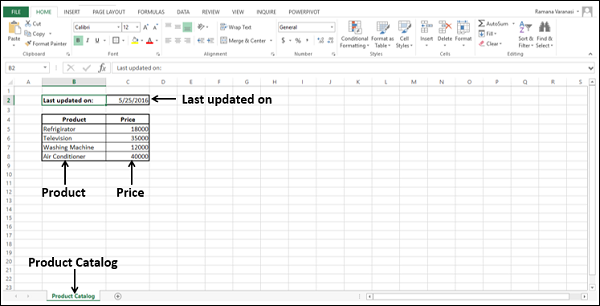## Multiple Worksheets with same Structure

Next, you have to set up worksheets for the Regions – East, North, South, and West, in that order with same structure.

• Name the worksheets East, North, South and West.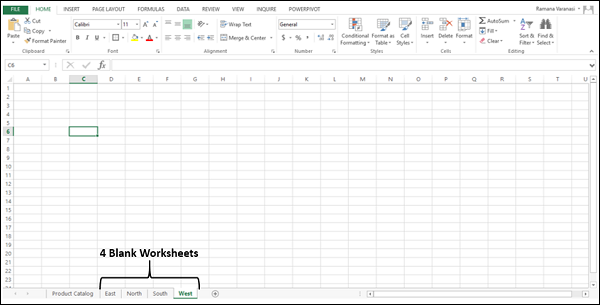These four worksheets should have the same structure.

• Click the tab East. The East worksheet opens.
• Press the shift key and click on the tab West. All the 4 tabs will be selected.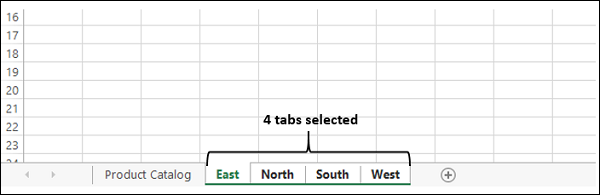Now, whatever editing you do in the East worksheet will get automatically reflected in the other three selected worksheets.

In the East worksheet,

• Add the column headers – S. No., Month, Product, Price, No. of Units, Total Amount.
• Add the S. No., Month April and the 4 Product Names.
• Format the Table.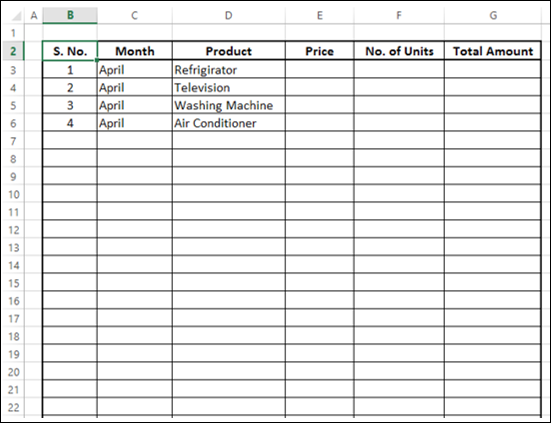The same structure appears in the other worksheets North, South and West.

## Creating a Formula across Multiple Worksheets

To create a formula across multiple worksheets −

• Define names for the price values of the products in the product catalog worksheet.
• Set the Scope as Workbook for all the names.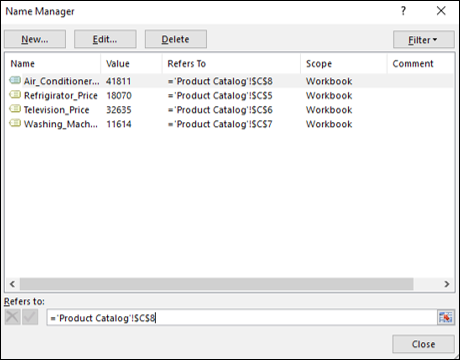• Once again select all the four worksheets – East, North, South and West.
• In the East worksheet, for each product, in the price column, give the formula as the Price Value Name.

As we defined earlier, the price of a product is as per the product catalog that is updated on the first of every month.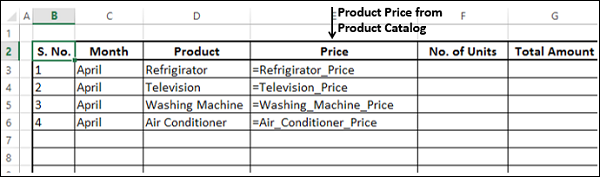• Repeat the same steps for each Month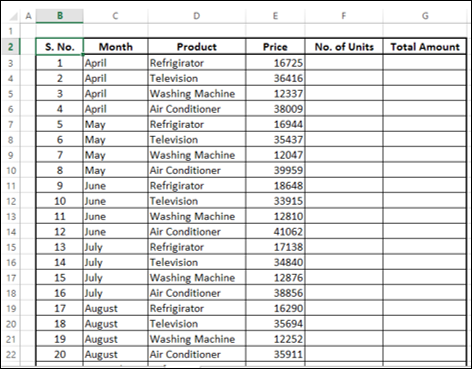Therefore, for the worksheets for the regions East, North, South, and West, you have successfully set the same structure and placed the price information for each product based on the month from product catalog worksheet.

The Product Catalog can be in another Workbook too.

### Calculations in the Worksheets

The next step is to fill in the information of No. of Units sold for each Product in each Month and in each Region. Therefore, you need to work separately on these worksheets.

For each region, for each product −

• Fill No. of Units sold.
• Calculate the corresponding Total Amount as Price*No. of Units.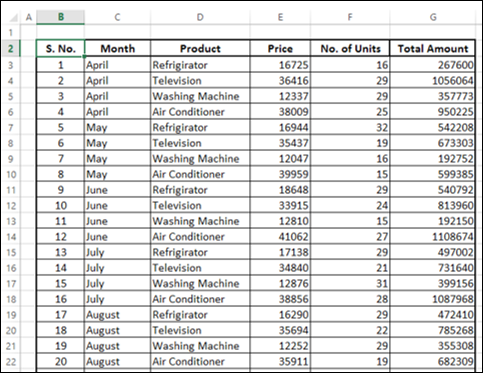In each worksheet (East, North, South and West), calculate subtotals month-wise −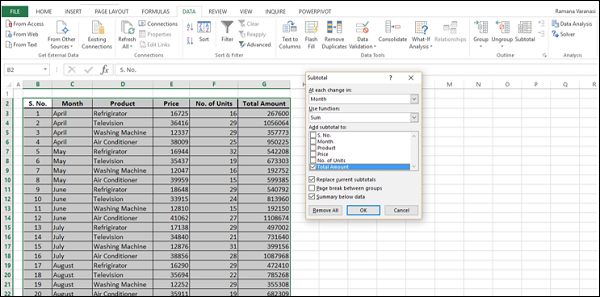Note − You can use Subtotal on a single worksheet but not on multiple worksheets. Hence, you have to repeat this step for North, South and West worksheets.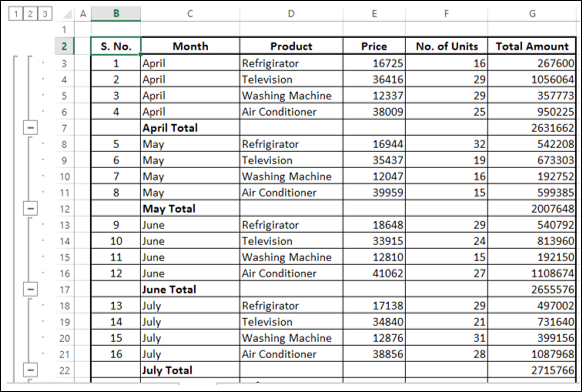Click the Outline Level 2. You will get all month-wise Totals.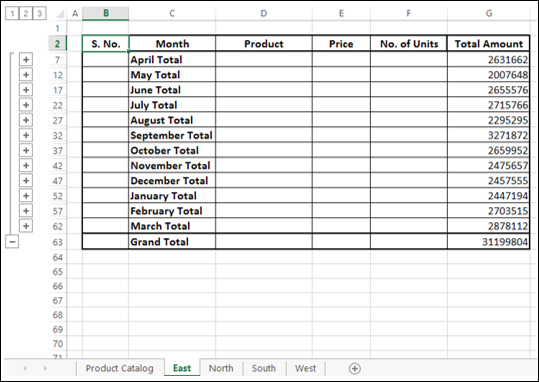Now, you are ready to summarize the results from all the four worksheets – East, North, South and West.

## Summarizing Data in Multiple Worksheets

The following steps will show you how to summarize data from multiple worksheets.

• Add a worksheet and name it Summary.
• Create the structure for Summary worksheet.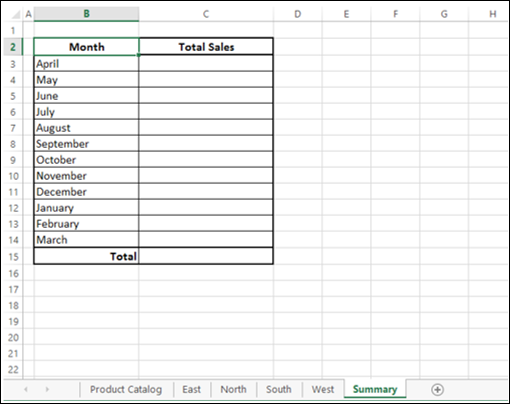In the column- Total Sales, in the cell C3, type =sum(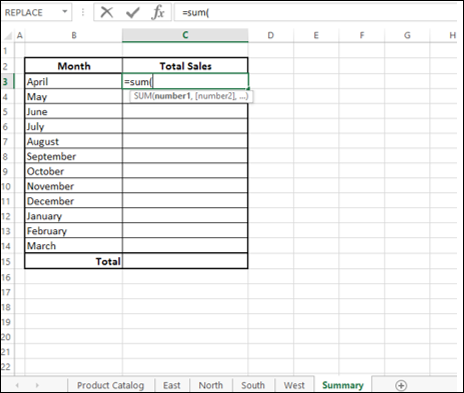• Select the worksheet East.
• Select the cell G7.
• With East tab pressed, click the tab West.
• The tabs East to West get selected.
• The formula in the formula bar appears as

=sum(‘East:West’!G7)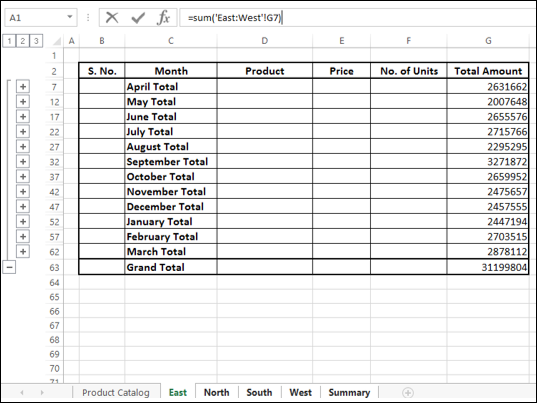Note that you are still in the East worksheet. Press Enter.

You will be in the Summary worksheet. In the formula bar, you will see the formula as

=SUM(East:West!G7)

The calculated value appears in the cell C3.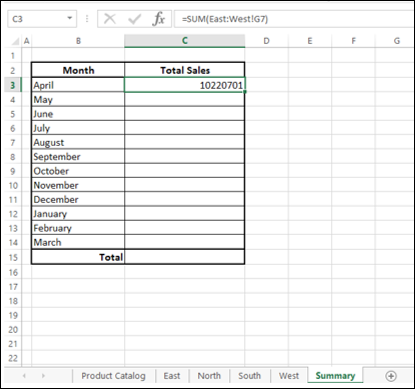• Copy the formula to the cells C4 to C14.
• Click Show Formulas in the Formula Auditing group under the FORMULAS tab.

All the formulas in the column Total Sales appear.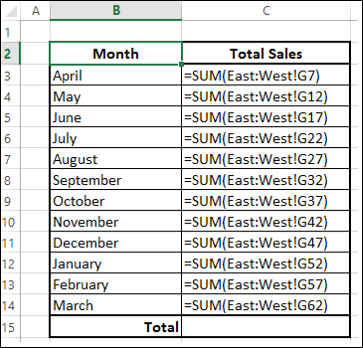This is exactly how you wanted to summarize the results from each region.

• Click in the cell C15.
• Type =sum(C3:C14)